# SSC CGL EXAMS 2018 | Quantitative Aptitude Practice Questions (Day-7)

Dear Aspirants, Here we have given the Important SSC Exam 2018 Practice Test Papers. Candidates those who are preparing for SSC 2018 can practice these questions to get more confidence to Crack SSC 2018 Examination.

[WpProQuiz 4144]

1) For measuring temperature two different units C and F are used. At which temperature both will show same value if the relation between then is.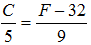a) 0o

b) 100o

c) –40o

d) 40o

2) 24 men and 6 women can do a work in 20 days. How much time it will take for 8 men and 2 women to complete 1/6th of the job?

a) 10 days

b) 20 days

c) 30 days

d) Cannot be determined

3) Sachin’s average score for 49 matches was 48 runs. How much he should score in his 50th match to make the average score ‘50’?

a) 50

b) 48

c) 148

d) 98

4) If a + (1/ (a – 4)) = 4 then find the value of (a –4)2 +1/ (a – 4)2.

a) –4

b) –2

c) 2

d) 4

5)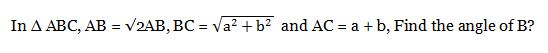a) 60o

b) 30o

c) 45o

d) 90o

6) Two trains running in opposite directions speed of 60 km/h and 90 km/h crosses each other in 30 sec. If the length of train 1 is 200 meters. Find the length of train?

a) 1200 meters

b) 800 meters

c) 750 meters

d) 1050 meters

7) Sameer invested a sum of money at compound interest. Maturity amount after 3 years is Rs. 8000 and amount after 4 years is Rs. 8400. The rate of interest per annum is:

a) 2 ½ %

b) 5%

c) 10%

d) 20%

8) How many common tangents can be drawn on two circles touching each other external?

a) Infinite

b) 2

c) 0

d) 3

9) The number of coins of diameter 1.5 cm and thickness 0.4 cm required to be melted to make a right circular cylinder of height 8 cm and base radius 3 cm is.

a) 320

b) 180

c) 280

d) 230

10) A conical vessel of radius 7 cm has height of 20 cm. It is filled upto 60% of height by water. How much volume water (in cubic cm) is filled in it?

a) 1848

b) 616

c) 1232

d) 924

11) ‘P’ is faster than ‘Q’. ‘P’ and ‘Q’ each walk 12 km. The sum of their speed is 7 km/h and the sum of times taken by them is 7 hours. Then P’s speed is equal to.

a) 3 km/h

b) 4 km/hr

c) 5 km/h

d) 7 km/h

12) A man buys a car for Rs. 2, 50,000 in cash and sells at Rs. 2, 85,000 in credit of 1 year. If the rate of interest is 10% per annum, then how much profit or loss will he have?

a) Rs. 10000 profit

b) Rs. 25000 profit

c) Rs. 10000 loss

d) Rs. 25000 loss

13) Find the Area of below part circular land of radius 28 cm.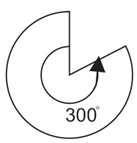a) 2464 cm2

b) 410.66 cm2

c) 1232 cm2

d) 2053.33 cm3

14)  If tan θ + (1/ tan θ) = 2 then the value of 2 sin2 θ + 4 cos2 θ = ?

a) 1

b) 2

c) 4

d) 0

15) Mohan is twice as old as vivek was 2 years ago. If the difference between their ages is 2 years, how old is Mohan today?

a) 6 years

b) 8 years

c) 10 years

d) 12 years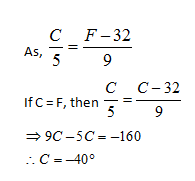24 men and 6 women can do work in 20 days.

24 men and 6 women do 1 work in 20 days

8 men and 2 women do 1 work in 203 days

8 men and 2 women do 1/6th work in 20 * 3 / 6 days

= 10 days.

Total score of 49 matches

= 49  48 = 2352

Total score in 50 matches for

Average 50 = 50  50 = 2500

Required score for 50th match = 2500 – 2352 = 148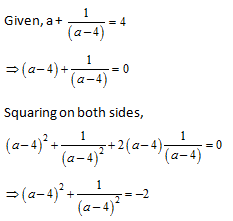All sides are side of right angle triangle and follow Pythagoras theorem: p2 + b2 = h2, Hence,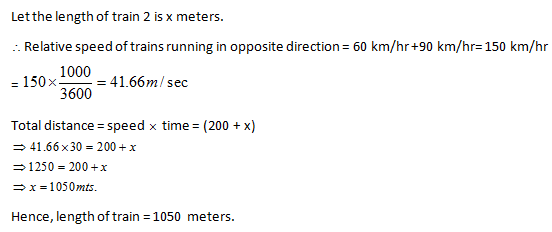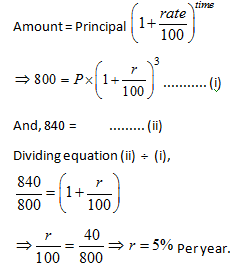SOLUTION: Three tangents: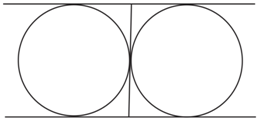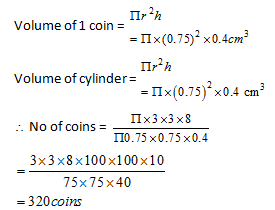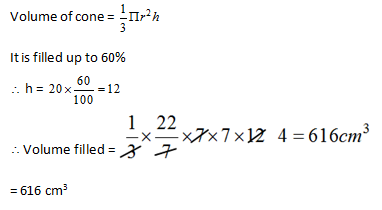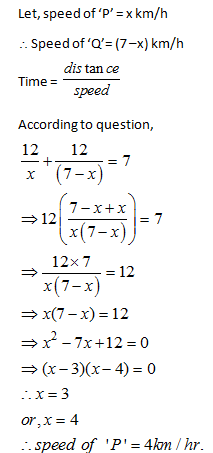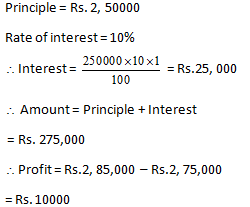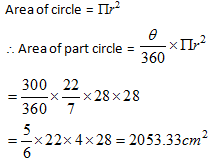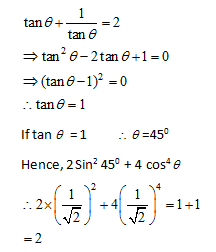Let, vivek’s age two years ago

Was x year

Mohan current age = 2x

According to question,

2x – (x+2) = 2

X – 2 = 2

=> 4 years

Current age of Mohan = 8 years

### SSC Quantitative Aptitude Practice Questions (Day-4)